Partitioning

Partitioning allows you to distribute all the data from a set into distinct subsets according to the rule applied on a table or row, where these subsets form a partition of the original set with no item replicated into multiple groups.

Partitioning data is an important feature to scale your Hop pipelines up and out. Scaling up makes the most of a single server with multiple CPU cores, while scaling out maximizes the resources of multiple servers operating in parallel.

Data Partitioning During Processing

By default, each transform in a pipeline is executed in parallel in a single separate thread.
Consider, for example, the pipeline below. With a single copy of each transform, the data is read from the CSV file input transform and then aggregated in the count by state transform. The results of which can be verified by examining the preview data.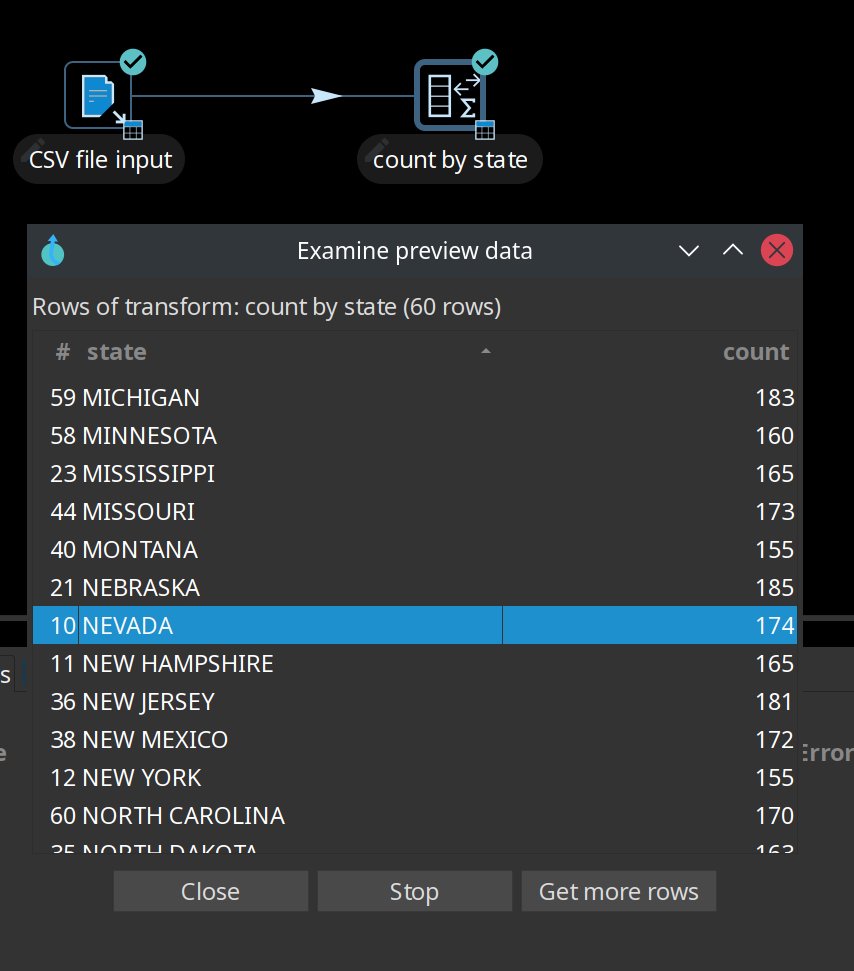To take advantage of the processing resources in your run configuration, you can scale up the pipeline using the multi-threading option Change Number of Copies to Start to produce multiple copies of the transform (click the transform’s icon to open the context dialog and select 'Specify copies' in the 'Data routing' category).

As shown below, the x2 notation indicates that two copies will be started at runtime.

By default, this data movement from the CSV file input transform into the count by state transform will be performed in round-robin order. This means that if there are 'N' copies, the first copy gets the first row, the second copy gets the second row, and the Nth copy receives the Nth row. Row N+1 goes to the first copy again, and so on until there are no more rows to distribute.

Reading the data from the CSV file is done in parallel. Attempting to aggregate in parallel, however, produces incorrect results because the rows are split arbitrarily (without a specific rule) over the two copies of the count by state aggregation transform, as shown in the preview data.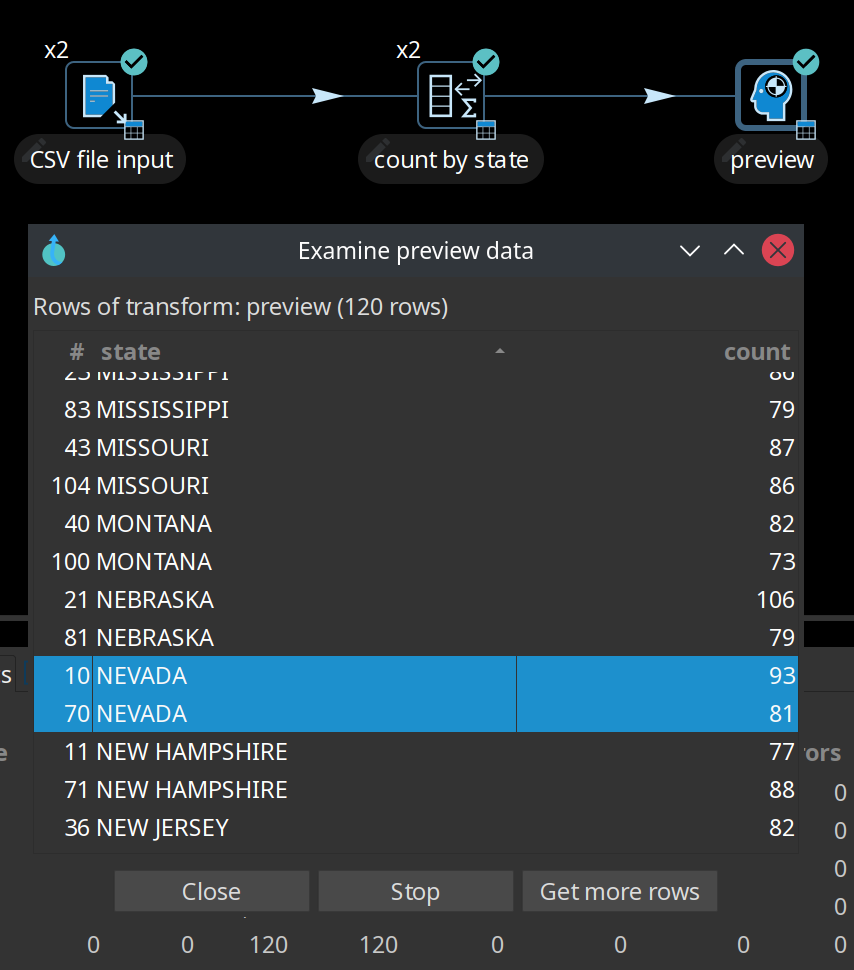Understand repartitioning logic

Data distribution in the transforms is shown in the following table.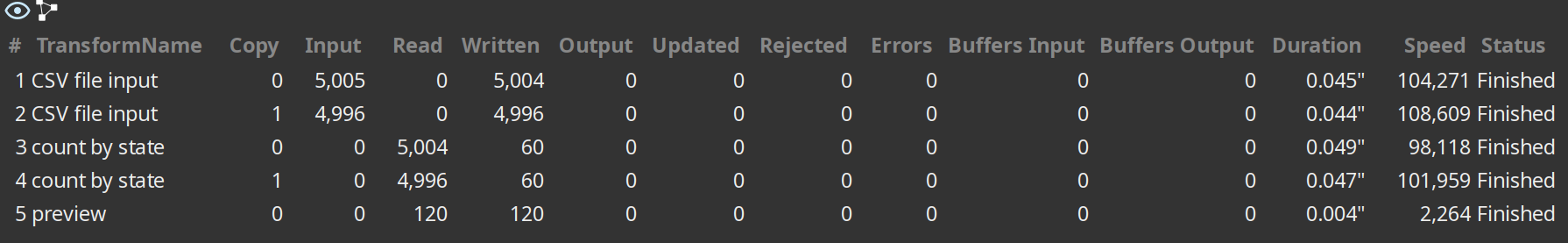As you can see, the CSV file input transform divides the work between two transform copies and each copy reads 5000 rows of data. However, these 2 transform copies also need to make sure that the rows end up on the correct count by state transform copy where they arrive in a 5004/4996 split. Because of that, it is a general rule that the transform performing the repartitioning (row redistribution) of the data (a non-partitioned transform before a partitioned one) has internal buffers from every source transform copy to every target transform copy, as shown below.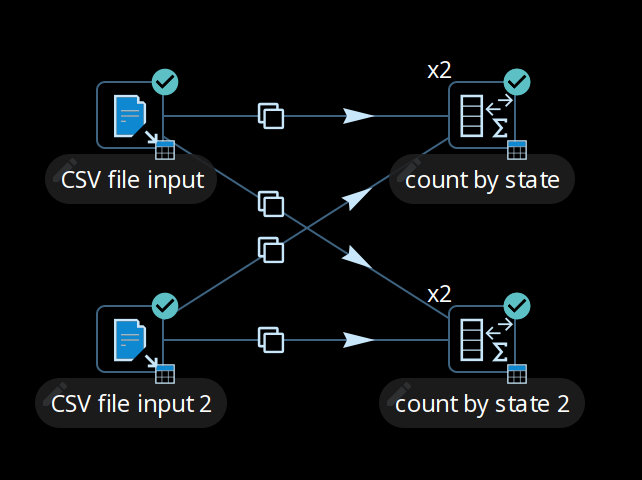This is where partitioning data becomes a useful concept, as it applies specific rule-based direction for aggregation, directing rows from the same state to the same transform copy, so that the rows are not split arbitrarily. In the example below, a partition schema called State was applied to the count by state transform and the Remainder of division partitioning rule was applied to the State field. Now, the count by state aggregation transform produces consistent correct results because the rows were split up according to the partition schema and rule, as shown in the preview data.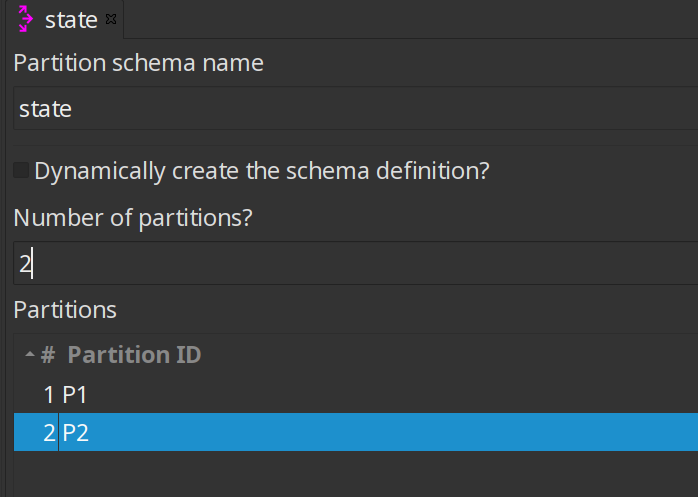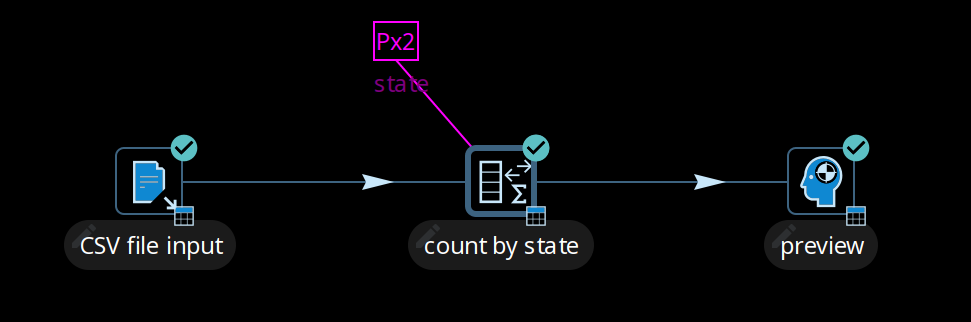Partitioning data over tables

The Table output transform supports partitioning rows of data to different tables. When configured to accept the table name from a Partitioning field, the PDI client will output the rows to the appropriate table. You can also Partition data per month or Partition data per day. To ensure that all the necessary tables exist, we recommend creating them in a separate pipeline.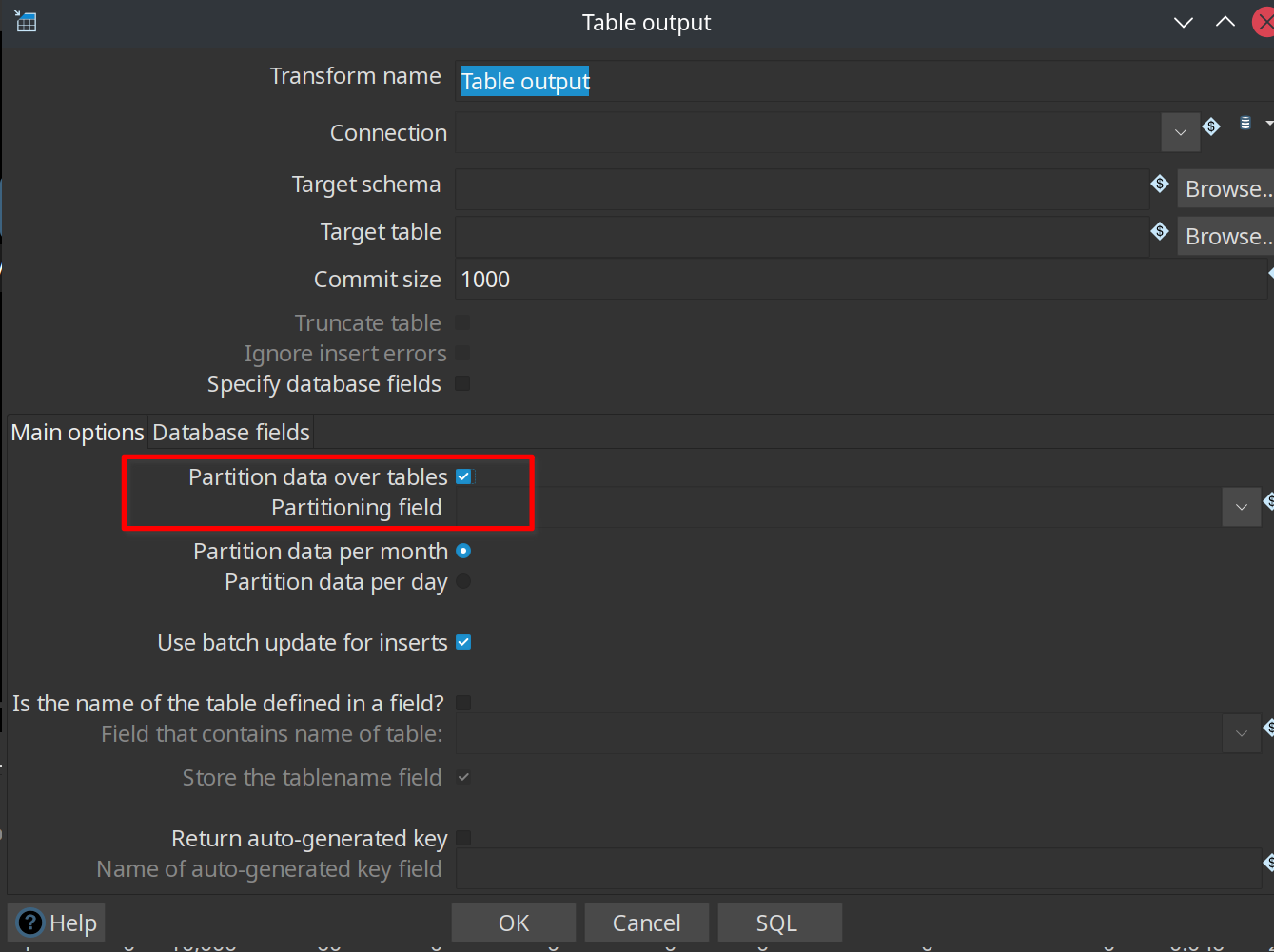Use partitioning

The partitioning method you use can be based on any criteria, can include no rule (round-robin row distribution), or can be created using a partitioning method plugin. The idea is to establish a criterion by which to partition the data, so that resulting storage and processing groups are logically independent from each other.

Step One: setup the partition schema:

1. First, configure a partition schema. A partition schema defines how many ways the row stream will be split. The names used for the partitions can be anything you like.

2. Next, apply the partition schema to the Group By transform. By applying a partition schema to a transform, a matching set of transform copies is started automatically (for example, if applying a partition schema with three partitions, three transform copies are launched).

Step Two: select the partitioning method:

• Establish the partitioning method for the transform, which defines the rule for row distribution across the copies. The Remainder of division rule allows rows with the same state value to be sent to the same transform copy and the distribution of similar rows among the transforms. If the modulo is calculated on a non-integer value, the PDI client calculates the modulo on a checksum created from the String, Date, and Number value.

 When you run the pipeline, there are no guarantees as to which page name goes to which transform copy, only that any page name encountered is consistently forwarded to the same transform copy.

Use data swimlanes

When a partitioned transform passes data to another partitioned transform with the same partition schema, the data is kept in swimlanes because no repartitioning needs to be done. As illustrated below, no extra buffers (row sets) are allocated between the copies of transforms count by state and Replace in string.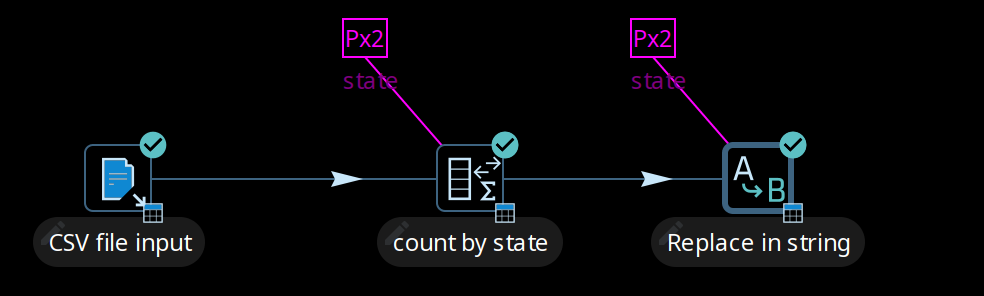The transform copies remain isolated from one another and the rows of data travel in swimlanes. No extra work needs to be done to keep the data partitioned, so you can chain as many partitioned transforms as needed. This will internally be executed as shown in the following illustration.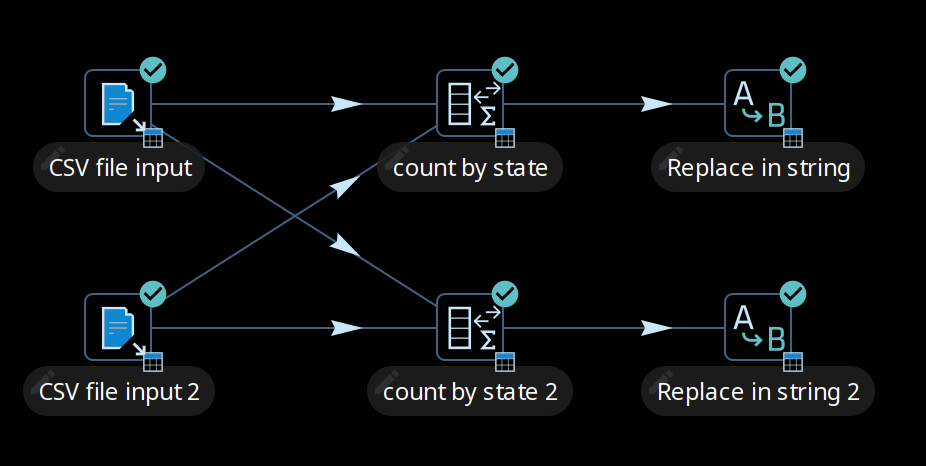Rules for partitioning

When you use partitioning, the logic used for distribution, repartitioning, and buffer allocations will be dependent upon the following rules:

• A partitioned transform causes one transform copy to be executed per partition in the partition schema.

• When a transform needs to repartition the data, the transform creates buffers (row sets) from each source transform copy to each target transform copy (partition).

• When rows of data pass from a non-partitioned transform to a partitioned one, data is repartitioned and extra buffers are allocated.

• When rows of data, partitioned with the same partition schema, pass from a partitioned transform to another partitioned transform, data is not repartitioned.

• When rows of data, partitioned with a different partition schema, pass from a partitioned transform to another partitioned transform, data is repartitioned.